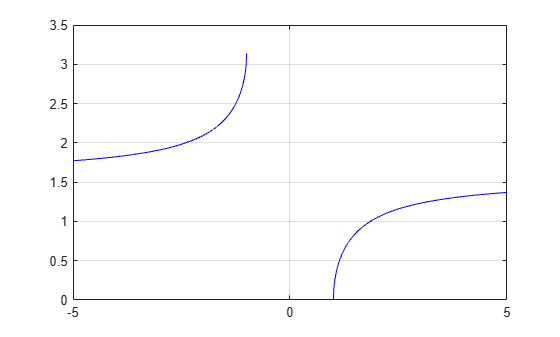# asec

## Syntax

``Y = asec(X)``

## Description

example

````Y = asec(X)` returns the Inverse Secant (sec-1) of the elements of `X` in radians. The function accepts both real and complex inputs. For real values of `X` in the interval [-∞, -1] and [1, ∞], `asec(X)` returns values in the interval [0, π].For real values of `X` in the interval (-1, 1) and for complex values of `X`, `asec(X)` returns complex values. ```

## Examples

collapse all

Find the inverse secant of a value.

`asec(-2.8)`
```ans = 1.9360 ```

Find the inverse secant of the elements of vector `x`. The `asec` function acts on `x` element-wise.

```x = [0.5i 1+3i -2.2+i]; Y = asec(x)```
```Y = 1×3 complex 1.5708 + 1.4436i 1.4749 + 0.2970i 1.9503 + 0.1833i ```

Plot the inverse secant function over the intervals $-5\le x\le -1$ and $1\le x\le 5$.

```x1 = -5:0.01:-1; x2 = 1:0.01:5; plot(x1,asec(x1),'b') hold on plot(x2,asec(x2),'b') grid on```## Input Arguments

collapse all

Secant of angle, specified as a scalar, vector, matrix, multidimensional array, table, or timetable. The `asec` operation is element-wise when `X` is nonscalar.

Data Types: `single` | `double` | `table` | `timetable`
Complex Number Support: Yes

collapse all

### Inverse Secant

The inverse secant is defined as

`${\mathrm{sec}}^{-1}\left(z\right)={\mathrm{cos}}^{-1}\left(\frac{1}{z}\right).$`

## Version History

Introduced before R2006a

expand all